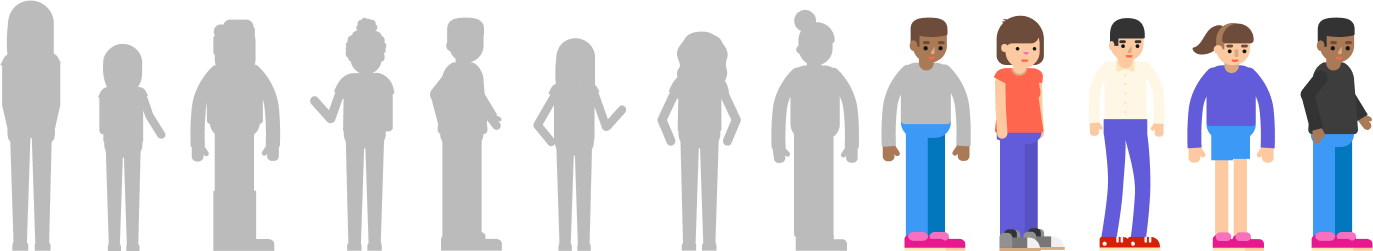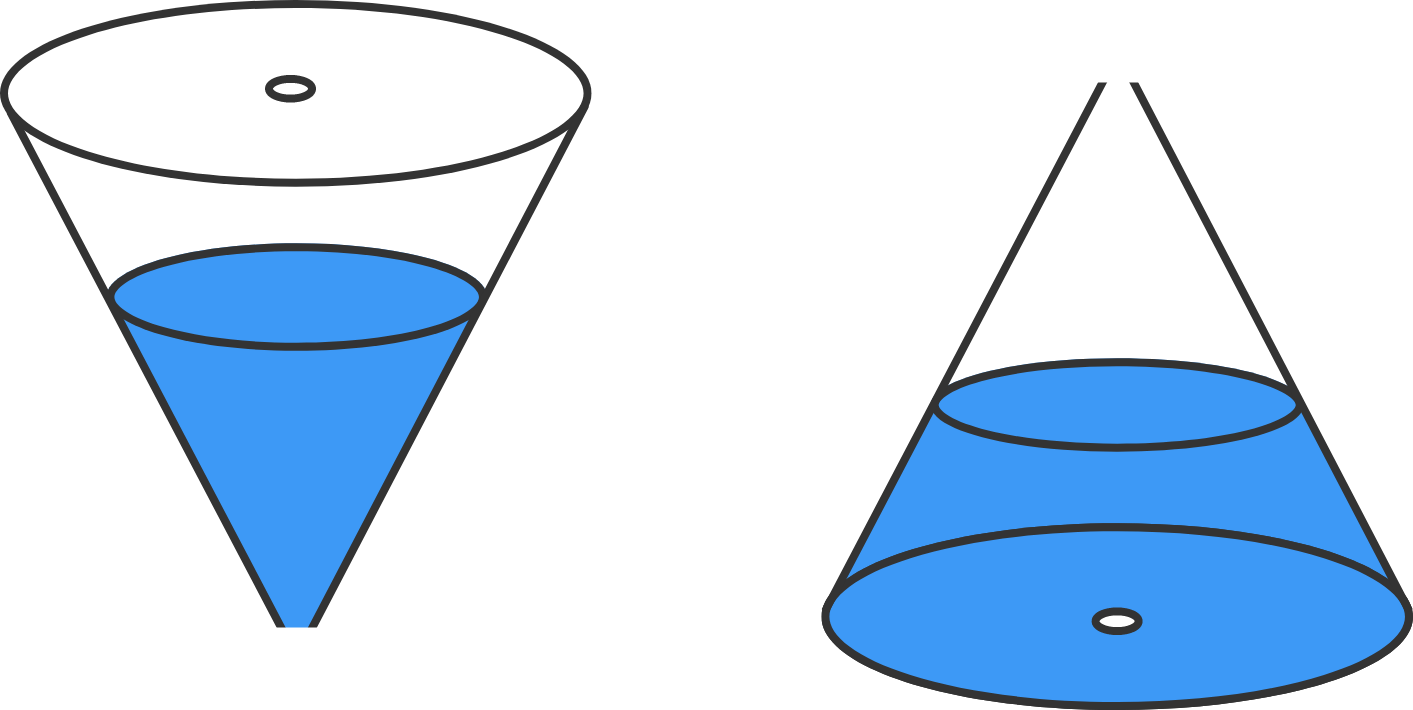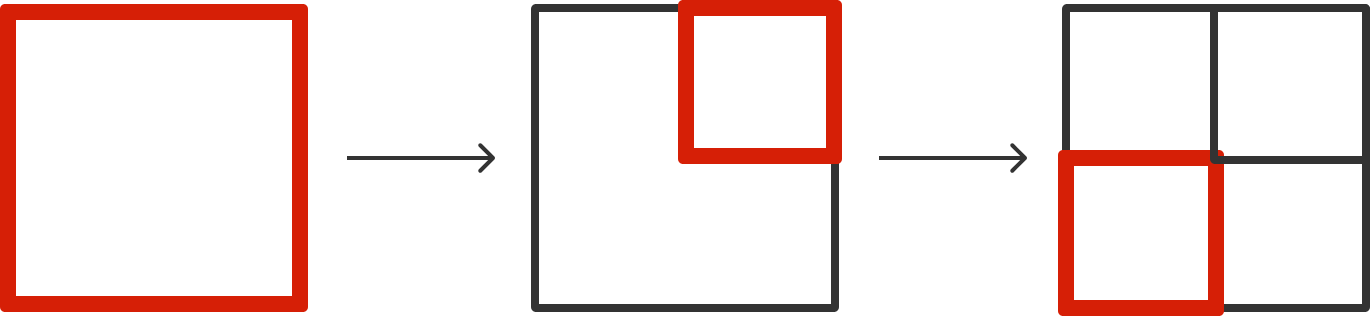# Problems of the Week

Contribute a problem

# 2018-01-08 Basic

Some students, including Rohit and Pranshu, are standing in a line.

• Rohit is the 5th person in line counting from the right.
• Pranshu is the 5th person in line counting from the left.
• There are 3 people in the line between Pranshu and Rohit.

How many people are there in the line?True or False?

If $x$ is a real number satisfying $\frac{1}{x} < 10,$ then it must be true that $x > \frac{1}{10}.$

Each cone below has two holes: one at the apex and another in the base. They differ only in that the cone on the left is turned upside down.

If both cones initially contain the same amount of water and if they begin to drain simultaneously, which cone will take less time to empty out?Each of the four distinct keys below fits exactly one of the four locks. In the worst case scenario, how many times do you have to test a key in a lock in order to match up all the locks and keys?Note: You do not need to open the locks. You only need to match the locks to their keys.

As illustrated below, Alice needs to draw just 3 (red) squares to create 4 unit squares.

What is the least number of squares she needs to draw to create 16 unit squares?×

Problem Loading...

Note Loading...

Set Loading...